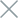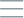• Home
• Tags
• Series
•# Greedy Algorithm in Java: Explained with Examples

The Greedy Algorithm is an optimization technique in Java programming characterized by selecting the best solution at each step without revisiting or considering the future. Instead of examining the entire state space, this algorithm chooses the best current option and hopes that this will lead to a global optimal solution.

## How the Greedy Algorithm Works

1. Step 1: Start from the initial state.

2. Step 2: At each step, the algorithm selects the best option among the available options based on an evaluation function.

3. Step 3: The algorithm moves to a new state by choosing the best option.

4. Step 4: The process continues until a termination condition is met or there are no more options to choose from.

5. Step 5: Return the found solution.

• Simplicity: Easy to understand and implement.
• Efficiency: Often requires less computation time and memory compared to some other optimization algorithms.
• Ideal for suboptimal problems: Suitable for problems where considering all possibilities is too complex.

• No global optimal guarantee: The algorithm may stop at a local optimal solution without finding the global optimal one.
• Lack of foresight: The algorithm often doesn't consider the consequences of previous decisions.

## Example and Explanation

A common example of the Greedy Algorithm is finding the "Kth Largest Element" problem. Let's see how this algorithm works:

``````import java.util.Arrays;

public class GreedyAlgorithmExample {
static int findKthLargest(int[] nums, int k) {
Arrays.sort(nums); // Sort the array
return nums[nums.length - k]; // Return the kth largest element
}

public static void main(String[] args) {
int[] nums = {3, 1, 2, 4, 5};
int k = 2;
int result = findKthLargest(nums, k);
System.out.println("The " + k + "th largest element is: " + result);
}
}
``````

In the above example, we use the Greedy Algorithm to find the second largest element in an array of integers. This algorithm simply sorts the array and returns the kth largest element. Although it's not guaranteed to be the global optimal, it's a relatively good solution for this problem.